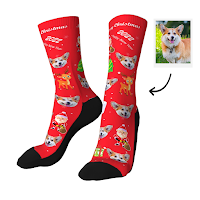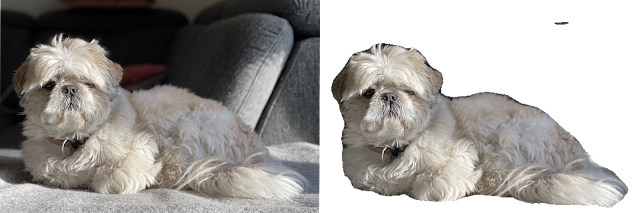### The idea

A while back I saw a youtube video of a guy who created a dropshipping website to sell custom socks with customers' dog pictures on them. During the ordering process, customers can upload their custom dogs, and backgrounds are removed by skilled artists on Fiverr. Images are printed on socks and shipped.
I wondered could we automate the background removal process, lets's give it a GO!

###Semantic image segmentation, enter Deeplab

Background removal is quite difficult, what constitutes the background and what is the subject? A simple algorithmic approach would not work.  Luckily advances in neural networks could be of use to us, after googling a bit, this showed some promise it can determine the background and the subject, just what we need!
Now to download the model and set up a go project, here is a great resource to get some inspiration.

Let's set up a GO project using TensorFlow GO en using the example to guide us

Neural nets are trained with fixed image sizes, our network happens to be 513(w)x513(h)x3(RGB) input size, so here is the resizing functionality :

`package imageimport (   "github.com/nfnt/resize"   "image"   "math")const InputSize = 513func ResizeImage(image image.Image) image.Image {   bounds := image.Bounds()   resizeRatio := 1.0 * InputSize /       math.Max(float64(bounds.Size().X), float64(bounds.Size().Y))   tWidth := uint(resizeRatio * float64(bounds.Size().X))   tHeight := uint(resizeRatio * float64(bounds.Size().Y))   return resize.Resize(tWidth, tHeight, image, resize.Bilinear)}`

`// loading modelmodel, err := ioutil.ReadFile(modelPath)if err != nil {   log.Fatal(err)}graph := tf.NewGraph()if err := graph.Import(model, ""); err != nil {   log.Fatal(err)}// Create a session for inference over graph.session, err := tf.NewSession(graph, nil)if err != nil {   log.Fatal(err)}defer session.Close()`
TensorFlow groks TensorFlow structs so we have to make one (gleaned from the example resources :-)

`// Convert the image in filename to a Tensor suitable as input to the Inception model.func makeTensorFromImage(m image.Image) (*tf.Tensor, error) {   buf := new(bytes.Buffer)   err := jpeg.Encode(buf, m, nil)   if err != nil {      return nil, err   }   // DecodeJpeg uses a scalar String-valued tensor as input.   tensor, err := tf.NewTensor(string(buf.Bytes()))   if err != nil {      return nil, err   }   // Construct a graph to normalize the image   graph, input, output, err := constructGraphToImage()   if err != nil {      return nil, err   }   // Execute that graph to normalize this one image   session, err := tf.NewSession(graph, nil)   if err != nil {      return nil, err   }   defer session.Close()   normalized, err := session.Run(      map[tf.Output]*tf.Tensor{input: tensor},      []tf.Output{output},      nil)   if err != nil {      return nil, err   }   return normalized, nil}`

When the tensor is made we can run the TF session:
`output, err := session.Run(   map[tf.Output]*tf.Tensor{      graph.Operation("ImageTensor").Output(0): tensor,   },   []tf.Output{      graph.Operation("SemanticPredictions").Output(0),   },   nil)`
the resulting segmentation image looks like this with a random color pallet :

the segmentation has 3 parts 0(black), 18(white), 12(green), according to the label index dummy, Badger, Persian cat(Binky would disagree), but for this task masking values of 0 to 0 and > 0 to 255 alpha.
`shape := output.Shape()zeroImg := image.NewAlpha(image.Rect(0, 0, int(shape), int(shape)))rgbImage := output.Value().([][][]int64)for y := 0; y < len(rgbImage)-1; y++ {   for x := 0; x < len(rgbImage[y])-1; x++ {      if rgbImage[y][x] == 0 {         zeroImg.SetAlpha(x, y, color.Alpha{uint8(0)})      } else {         zeroImg.SetAlpha(x, y, color.Alpha{uint8(255)})      }   }}`
After resizing the mask to the original image size and applying it to the original image:
`endresult := image.NewRGBA64(image.Rect(0, 0, w, h))for x := 0; x < w; x++ {   for y := 0; y < h; y++ {      alphaColor := segmentedImage.At(x, y)      imageColor := dog.At(x, y)      rr, gg, bb, _ := imageColor.RGBA()      _, _, _, alpha := alphaColor.RGBA()      endresult.Set(x, y, color.NRGBA64{uint16(rr), uint16(gg), uint16(bb), uint16(alpha)})   }}`

### End result

Here are some of the results not perfect but pretty neat! now to set up a million \$ business and sell some socks, but I let that up to you to make that happen! here is the github code, I uploaded the smallest TF model, the results depicted below are of the deep lab models > 400 MB!Binky - Shih Tzu(not a cat)

it works also on larger dogs ;-)